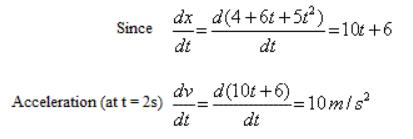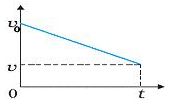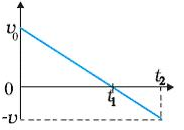Courses

# Test: Acceleration

## 10 Questions MCQ Test Physics Class 11 | Test: Acceleration

Description
This mock test of Test: Acceleration for Class 11 helps you for every Class 11 entrance exam. This contains 10 Multiple Choice Questions for Class 11 Test: Acceleration (mcq) to study with solutions a complete question bank. The solved questions answers in this Test: Acceleration quiz give you a good mix of easy questions and tough questions. Class 11 students definitely take this Test: Acceleration exercise for a better result in the exam. You can find other Test: Acceleration extra questions, long questions & short questions for Class 11 on EduRev as well by searching above.
QUESTION: 1

### A boy throws up a ball in a stationary lift and the ball returns to his hands in 10 s. Now if the lift starts moving up at a speed of 5 m/s. The time taken for a ball thrown straight up to return to his hands is:

Solution:

Lift moving with Uniform speed  = 5m / s

Ball is thrown inside the lift

The uniform velocity of the lift does not affect the relative velocity of the ball with respect to the boy.

Relative velocity of ball = 49m/s

⇒ Relative acceleration = 9.8

Thus, u(relative) = velocity of bell

t = 2u/ gr

​= (2 × 49) / 9.8

⇒ t = 10s

so, the ball will still return in 10 secs

QUESTION: 2

### The displacement of a particle along x-axis is given by x = 4 + 6t + 5t2. Its acceleration at t = 2s

Solution:QUESTION: 3

### On acceleration-time graph, a horizontal line indicates:

Solution:

The horizontal line on any graph indicates that its value is not changing with respect to changing time if we are taking time on the x-axis and taking any physical quantity such as velocity, speed, acceleration on the y-axis and if the graph shows straight horizontal line means its value remains same w.r.t time hence its value is constant.

QUESTION: 4

The position x of a particle varies with time (t) as x = 3t2 – 2t3  .The acceleration of the particle will be zero at time

Solution:

We know that acceleration is the second derivative of change in displacement wrt time, hence we get a = 6 - 12t
When a = 0 = 6 -12t ;
We get t = ½

QUESTION: 5

What does this graph indicate about the motion of an object ?Solution:

Velocity is decreasing so, acceleration is negative. Velocity is positive so direction is positive.

Hence option (C) is correct.

QUESTION: 6

The dimensions of (change in velocity / time) is

Solution:

We know that change in velocity / time is acceleration.

And dimension of acceleration is LT-2

QUESTION: 7

The dimensions of instantaneous acceleration is:

Solution:

Instantaneous acceleration is rate of change of instantaneous velocity with time or velocity upon total time, hence we get its dimension is same as of acceleration i.e. LT-2

QUESTION: 8

What does this graph indicate about the motion of an object?Solution:

Through the given graph we get that:
Velocity is decreasing from a positive constant to some negative number with zero at t1

Distance from the original point increases from zero to t1 and then it decreases afterwards.

The object is moving in a positive direction at first and after time t1 it moves in a negative direction.

QUESTION: 9

On acceleration-time graph the area under the curve equals the:

Solution:

The area under an acceleration graph represents the change in velocity.

In other words, the area under the acceleration graph for a certain time interval is equal to the change in velocity during that time interval.

QUESTION: 10

If the signs of the velocity and acceleration of a particle are the same, the speed of the particle ______________.

Solution:

In this question, it has been asked about the signs of velocity and acceleration not about the values of velocity and acceleration.

So, if both the entities have same the signs, then the speed of the object will increase.

If it is accelerating in the positive direction, the negative velocity is decreasing, and the object is slowing down.